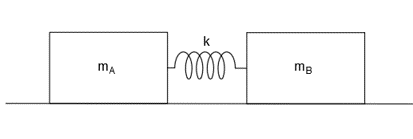# The masses mA=40 kg and mB=30 kg and spring constant k=400 N/m. The two masses are released from...

## Question:

The masses m{eq}_A {/eq}=40 kg and m{eq}_B {/eq}=30 kg and spring constant k=400 N/m. The two masses are released from rest on the smooth surface with the spring stretched 1 m.

What are the magnitudes of the velocities of the masses when the spring is unstretched?## Spring

Spring is used to minimize the vibrations in cars, machines, etc. It absorbs the mechanical energy and releases it when required. The springs are used in the clutches also.

## Answer and Explanation: 1

Become a Study.com member to unlock this answer! Create your account

Given Data

• The mass of block A is: {eq}{m_A} = 40\;{\rm{kg}} {/eq}.
• The mass of block B is: {eq}{m_B} = 30\;{\rm{kg}} {/eq}.
• The spring constant...

See full answer below.

#### Learn more about this topic:Practice Applying Spring Constant Formulas

from

Chapter 17 / Lesson 11
3.4K

In this lesson, you'll have the chance to practice using the spring constant formula. The lesson includes four problems of medium difficulty involving a variety of real-life applications.